StatLect

# Wald test

The Wald test is a test of hypothesis usually performed on parameters that have been estimated by maximum likelihood.

Before reading this lecture, the reader is strongly advised to read the lecture entitled Maximum likelihood - Hypothesis testing, which introduces the basics of hypothesis testing in a maximum likelihood (ML) framework.## The null hypothesis

In what follows, we are going to assume that an unknown parameterhas been estimated by ML, that it belongs to a parameter space, and that we want to test the null hypothesiswhereis a vector valued function, with. The aforementioned lecture on hypothesis testing gives some examples of common null hypotheses that can be written in this form.

## Assumptions

We are going to assume that the following technical conditions are satisfied:

• for each, the entries ofare continuously differentiable with respect to all entries of;

• thematrix of the partial derivatives of the entries ofwith respect to the entries of, called the Jacobian ofand denoted by, has rank.

## The Wald statistic

Letbe the estimate of theparameterobtained by maximizing the log-likelihood over the whole parameter space:whereis the likelihood function andis the sample.

Let us assume that the sample and the likelihood function satisfy some set of conditions that are sufficient to guarantee consistency and asymptotic normality of(see the lecture on maximum likelihood for a set of such conditions).

The test statistic employed in the Wald test is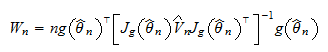whereis the sample size, andis a consistent estimate of the asymptotic covariance matrix of(see the lecture entitled Maximum likelihood - Covariance matrix estimation).

## The asymptotic distribution of the test statistic

Asymptotically, the test statistic has a Chi-square distribution, as stated by the following proposition.

Proposition Under the null hypothesis that, the Wald statisticconverges in distribution to a Chi-square distribution withdegrees of freedom.

Proof

We have assumed thatis consistent and asymptotically normal, which implies thatconverges in distribution to a multivariate normal random variable with meanand asymptotic covariance matrix, that is,Now, by the delta method, we have that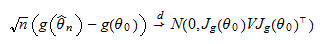But, so thatWe have assumed thatandare consistent estimators, that is,wheredenotes convergence in probability. Therefore, by the continuous mapping theorem, we have that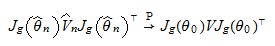Thus we can write the Wald statistic as a sequence of quadratic formswhereconverges in distribution to a normal random vectorwith mean zero, andconverges in probability to. By a standard result (see Exercise 2 in the lecture on Slutsky's theorem), such a sequence of quadratic forms converges in distribution to a Chi-square random variable with a number of degrees of freedom equal to.

## The test

In the Wald test, the null hypothesis is rejected ifwhereis a pre-determined critical value.

The size of the test can be approximated by its asymptotic value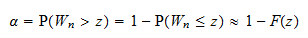whereis the distribution function of a Chi-square random variable withdegrees of freedom.

The critical valuecan be chosen so as to achieve a pre-determined size, as follows:## Example

The following example shows how to use the Wald test to test a simple linear restriction.

Example Let the parameter space be the set of all-dimensional vectors, i.e.,. Suppose we have obtained the following estimates of the parameter and of the asymptotic covariance matrix:whereis the sample size. We want to test the restrictionwhereanddenote the first and second component of. Then, the functionis a functiondefined byIn this case,. The Jacobian ofis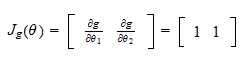which has rank. Note also that it does not depend on. We have thatAs a consequence, the Wald statistic is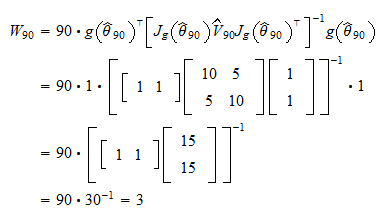Our test statistic has a Chi-square distribution withdegrees of freedom. Suppose we want our test to have size. Then, our critical valueis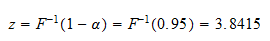whereis the distribution function of a Chi-square random variable withdegree of freedom and the value ofcan be calculated with any statistical software (for, example, in MATLAB with the command `chi2inv(0.95,1)`). Therefore, the test statistic does not exceed the critical valueand we do not reject the null hypothesis.

The book

Most of the learning materials found on this website are now available in a traditional textbook format.

Glossary entries
Share